# Graphs - Finding Minimum Spanning Trees with Kruskal's Algorithm

## a look into minimum spanning trees, kruskal's algorithm, and the cut property

I wrote this up to help solidify my own understanding of minimum spanning trees and Kruskal’s algorithm for the algorithms course I’m currently taking. Explaining things in my own words helps me learn and explaining it differently from what’s already out there may help someone else. 😌 In this post I’ll explain what minimum spanning trees are, include some examples, do a walkthrough of Kruskal’s algorithm, and try my best at explaining the cut property. At the end I’ll include some additional resources that I find helpful.

## What is a Minimum Spanning Tree

A minimum spanning tree of a weighted undirected graph is a subgraph that meets the following properties:

1. All vertices of the graph remain connected
2. There are no cycles in the graph
3. Total edge weight is minimized

Let’s consider the following graph G: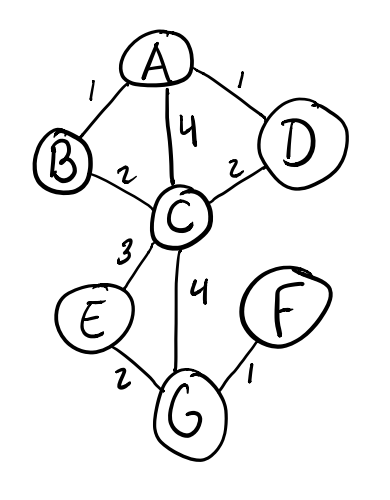As you can see, G is an undirected graph with weights on all edges. Now let’s look at some examples of subgraphs that are not minimum spanning trees.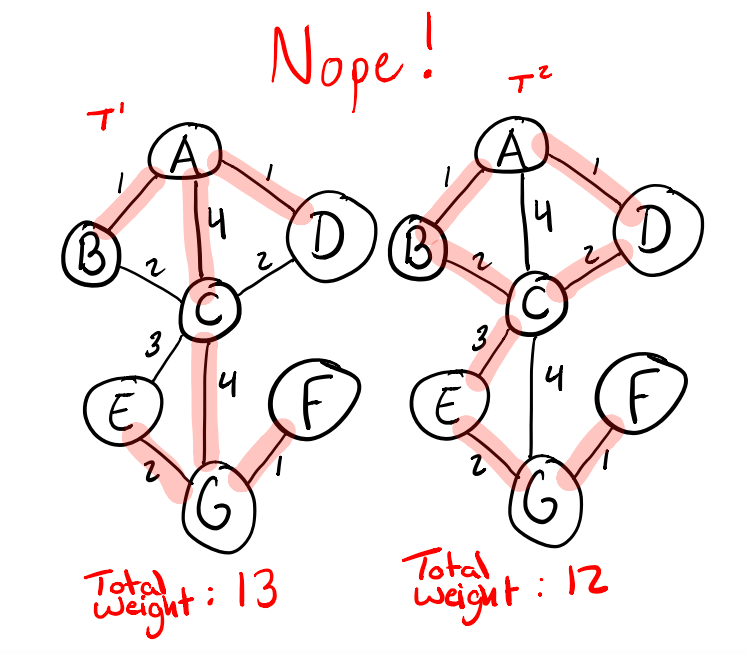First lets look at T1 on the left. Is this a spanning tree? Yes it is because the subgraph (denoted by lines highlighted in red) does not contain any cycles! Imagine you grabbed vertex C and let the other vertices hang freely. This might look like the following: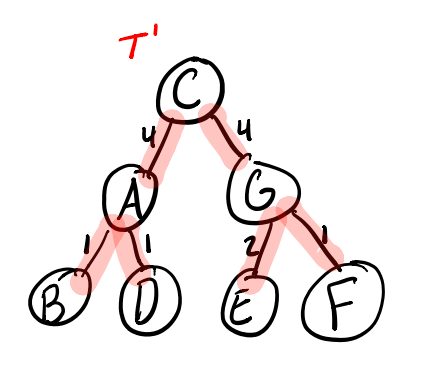See how it forms a tree structure?

By contrast, T2 is not a spanning tree because it contains a cycle between vertices A, B, C, and D. If we grab C on this one, notice how all the edges hang taut except for the cycular edges that tie B and D back to A.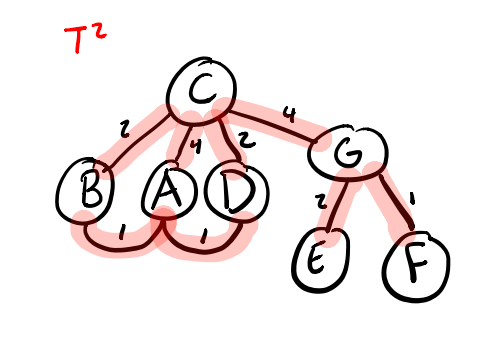So T2 is not a spanning tree so it clearly cannot be a minimum spanning tree. How come T1 isn’t? Well it violates our third property of minimizing total edge weight. T1 uses two of the most expensive edges of weight 4 to construct its tree when there are cheaper alternatives. So what do the minimum spanning trees for this G actually look like?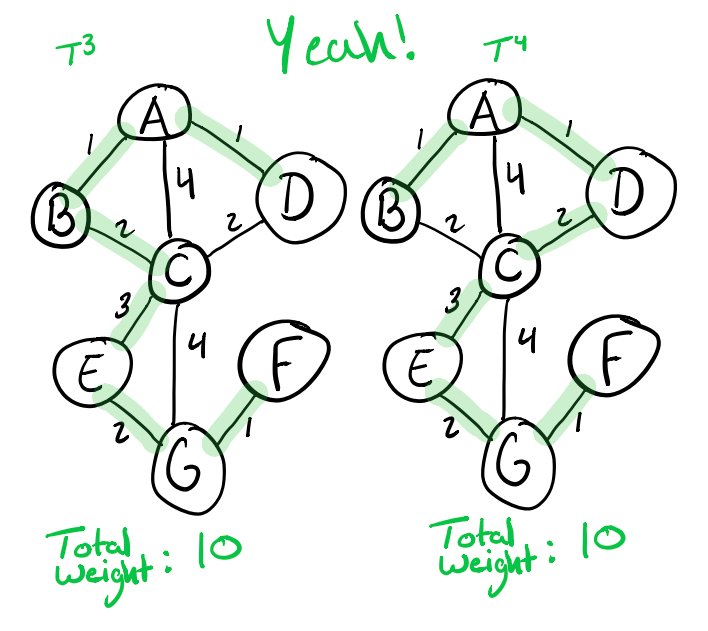Both T3 and T4 meet all of our criteria by connecting all of the vertices, not containing any cycles, and minimizing total edge weight. We can visualize the tree structure by doing the same exercise as before and hoisting up the C vertex for T3 as shown below: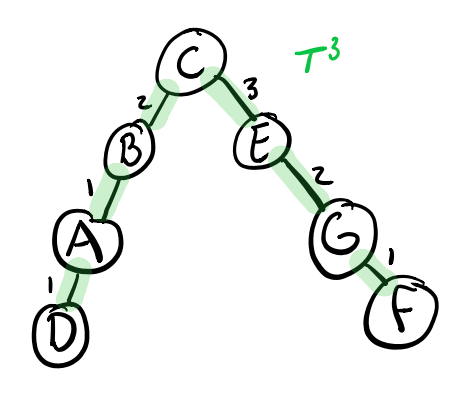That’s cool. So how can we programmatically find a minimum spanning tree from a graph? One way is by using Kruskal’s algorithm.

## Kruskal’s Algorithm for Finding Minimum Spanning Trees

Kruskal’s algorithm is a greedy algorithm, meaning that we’ll be making the most optimal choice locally for each subgraph as we work towards finding a minimum spanning tree for our graph G. We’ll be doing the following:

1. Sort the edges (E) of G by weight
2. Gradually add in edges, smallest first, to connect components without introducing a cycle
3. Repeat the process above until we run out of edges or only a single connected component remains (the minimum spanning tree). If we run out of edges then the graph is not connected so there is no minimum spanning tree for it.

Sounds pretty simple, right? The trickiest bit in my opinion is this portion: connect components without introducing a cycle. We can do this by using something called a union-find data structure. The linked video does a great job of explaining how it works, but in essence it will allow us to track which vertices are members of which connected components.

We’ll start by adding all vertices to the union-find as their own component, and as we add in edges we will gradually merge (or union) components. The way this will work is as follows:

1. When considering an edge e first do a find using the union-find data structure on the two vertices u and w connected by e. If find(u) and find(w) return the same result then both vertices are already members of the same component and adding this edge will introduce a cycle. In this case we cannot add e.
2. If find(u) and find(w) return different results then these two vertices are not currently connected and e is a valid candidate edge. We then perform union(u,v) on the vertices to union their components in the union-find. The next time we call find(u) and find(w) we will get the same result since they share a component.

Now assuming we have a sweet union-find at our disposal, let’s walk through Kruskal’s algorithm for our graph G.First let’s sort our edges by weight:

• A-B: 1
• A-D: 1
• F-G: 1
• B-C: 2
• D-C: 2
• E-G: 2
• E-C: 3
• A-C: 4
• C-G: 4

Now we’ll go over these edges in order, first looking at A-B.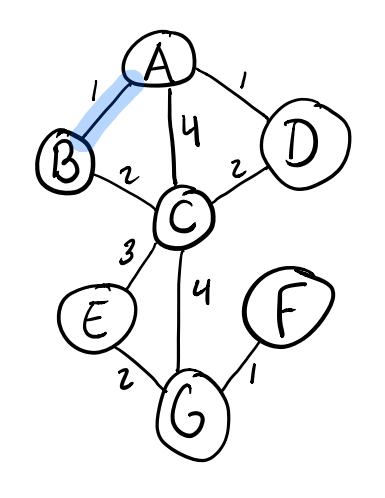It’s our first edge so there definitely won’t be a cycle here, but we’ll check our union-find just to be sure. find(A) and find(B) confirm our suspicions that these vertices are not connected yet, so we can union(A,B) and create our first connected component colored in blue.

Next we’ll look at A-D. We run find(A) and find(D) and see that these are separate components hence no cycle! Let’s add D to the blue component.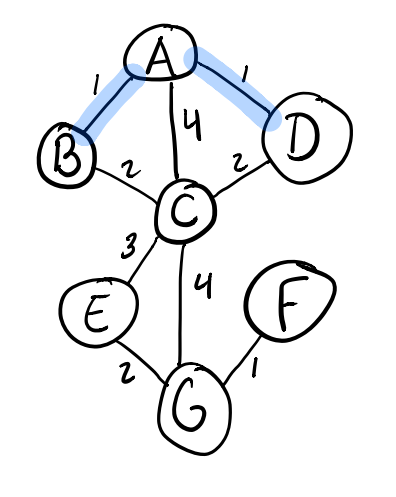Next we check out edge F-G and find(F) and find(G) shows that these two vertices are not currently connected. We run union(F,G) to form the orange component.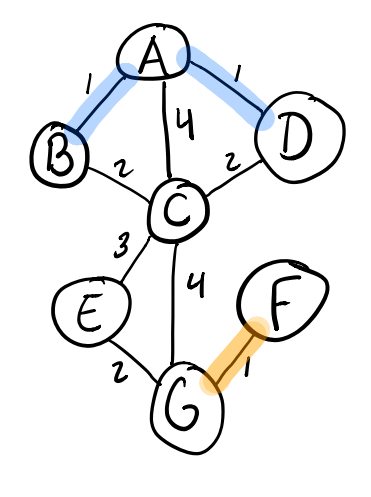Now we’re out of weight 1 edges so let’s move on to the weight 2 edges. We take a look at edge B-C and add it to the blue component that B is a member of using the same process as before.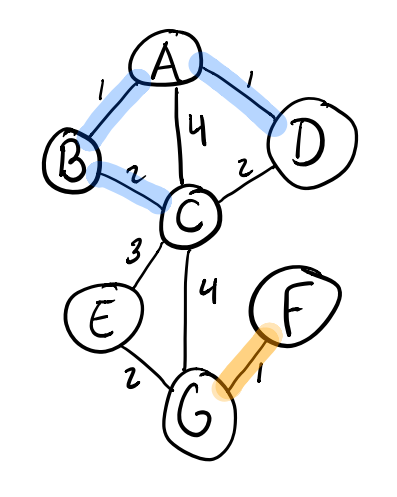Now let’s take a look at D-C. Here’s where things get a bit interesting. When we run find(D) and find(C) we get the same result for both since they’re both already members of the blue component. Adding in the edge from D-C will just add another way to connect these already connected vertices and introduce a cycle. Since spanning trees cannot contain cycles this is a no go.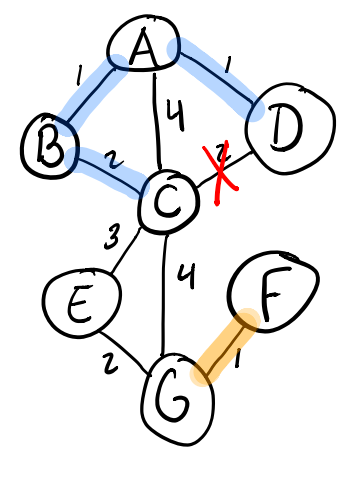Moving on to look at edge E-G we find that E and C are not already in the same component so we can union(E,G) and join them together as part of the orange component.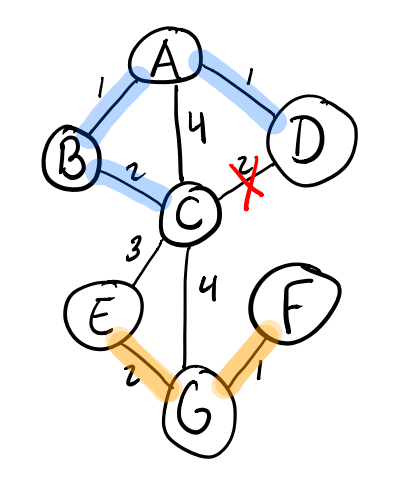We’ve now exhausted the weight 2 edges. Time to look at our weight 3 edge from E-C. If we run find(E) and find(C) we will see that these vertices are members of different components (orange and blue) so we can include the E-C edge to connect them. We’ve now joined our blue and orange components and have just a single component left! This means we’ve found our minimum spanning tree T3.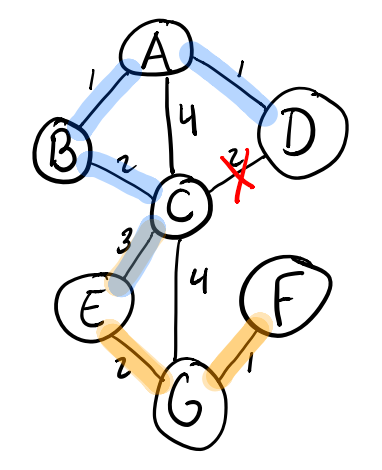## Cut Property

A cut of a graph is a partitioning of the graph into two groups S and the set of of vertices not in S (the complement of S), S̅. The image below shows what these cuts might look like for our graph G.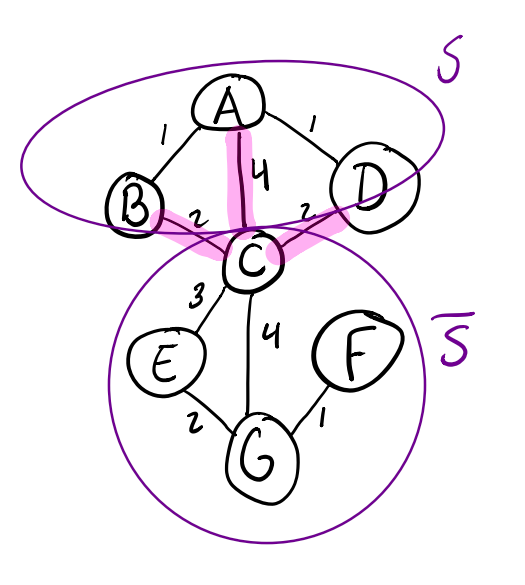The Cut Property states that any minimum weight edge across a cut must be part of some minimum spanning tree for the graph. You can kind of intuit this for our example. We can choose either the edge B-C or D-C (both are equal weight) and this will lead to one of our minimum spanning trees T3 or T4.

Erik Demaine’s lecture on Minimum Spanning Trees has a good proof of the Cut Property at around the 30-minute mark.